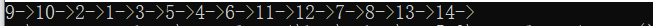# 有向无环图

``输入：G:一个顶点编号为从1~n的有向无环图; 输出：关于该图的一个拓扑序列; 步骤：     1.构造一个入度数组,in-degree，根据G，填充该入度数组; 	2.将入度数组中的每个入度值为0的顶点，压入next栈; 	3.只要next不为空，执行如下操作：         A.从next中弹出一个顶点u         B.将u添加到线性序列的末尾处         C.对于每个与u邻接的下游点v: 			i.令in-dgree[v]的值自减1             ii. 如果in-degree[v]=0，将v压入next栈中     4.返回线性序列 ````class Program     {       public static void Main()         {             var a = new Dictionary<int, List<int>> {  = new List<int> { 1 } };             var graph = new Dictionary<int, List<int>>             {                  = new List<int>{ 3 }, = new List<int>{4}, = new List<int>{4,5},                  = new List<int>{ 6 }, = new List<int>{ 6 },                  = new List<int>{ 7, 11 },                  = new List<int>{ 8 }, = new List<int>{13},                  = new List<int>{ 10 },                  = new List<int>{ 11 },  = new List<int>{ 12 },                  = new List<int>{ 13 },                  = new List<int>{14},=new List<int>()             };             var result = TopoloGicalSort(graph);             foreach (var re in result)                 Console.Write(re + "->");         }         /// <summary>         /// 用字典的形式来表示有向无环形图         /// 其中键是每个顶点，值是该顶点传向的顶点         /// </summary>         /// <param name="graph"></param>         public static List<int> TopoloGicalSort(Dictionary<int,List<int>>graph)         {             var result = new List<int>();             var inDegree = new Dictionary<int, int>();             //填充inDegree入度数组             foreach(var kv in graph)             {                 if (!inDegree.ContainsKey(kv.Key))                 {                     inDegree.Add(kv.Key, 0);                 }                 foreach(var verteice in kv.Value)                 {                     if (!inDegree.ContainsKey(verteice))                     {                         inDegree.Add(verteice, 1);                     }                     else                     {                         inDegree[verteice] += 1;                     }                 }             }             var next = new Stack<int>();//存放所有入度为0的顶点的栈             //next初始化             foreach(var kv in inDegree)             {                 if (kv.Value == 0)                 {                     next.Push(kv.Key);                 }             }             while (next.Count != 0)             {                 var verticeTemp = next.Pop();                 result.Add(verticeTemp);                 foreach (var vertice in graph[verticeTemp])                 {                     inDegree[vertice] -= 1;                     if (inDegree[vertice] == 0)                         next.Push(vertice);                 }             }             return result;         }     } ``### 推荐这些技术文章：

<2022年4月13号>

float:left
float:right

1.将块元素变成行内块，将行元素变成行内块

1.浮动
2.定位
3.弹性盒子

1.设置父级，设置好宽高

1.clear:both;在被影响的元素上加
2.给父级设置宽高加display:b...

foreach List<int>，List<string>怎么办？反之~

List<int> 跟 List<string> 是一一对应的吗？

...

C# list去重合并查找

1 List<int> listA = new List<int> { 1, 2, 3, 5, 7, 9 };
2 List<int> listB = new List<int> { 2,3,88 };
3 //合并
4 ...

maven 依赖冲突

<dependency>
<groupId>org.springframework.boot</groupId>
<artifactId>spring-boot-starter-oauth2-resource-server<...

c# list中的removeAll方法 两个List集合去除交集部分

static List<Object> list = new List<Object>() { 1,2,4,"a","b"};
static List<Object> list1 = new List<Object>() { 1, 2, 3,"a","c" };
static void Main(string[] ...

<P>&nbsp;</P>

...

Maven <build>

1. <build>

1.1 <resources>

1.2 <plugins>

Java 1.8 编译

Spring 打包

2. <properties>

1. <build>
1.1 <...

int i = 1 i >> 32或者i << 32都等于1,为什么?

int i = 1 i >> 32或者i << 32都等于1,为什么?请问有高手能详细回答下?

i >> n 和 i << n，在 n>=32的时候效果等同于n%32（这很好理解，因为i是int，那么移位操作位数最大就是31，如果这里是long的话那么最大就是63），因此...

THE END